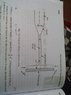# Fluid mechanics - impact of jet question

• patelsp004

#### patelsp004

Consider a case- Force excerted on a stationary flat plate held normal to the jet.

The Jet strikes on plate (see fig.) Which is smooth hence the exit velococity of jet = entering velocity of jet.

According to theory. The fore is applied on plate due to change in momentum of jet.

But my doubt is that since the jet have constant kinetic energy due to same velocity at entering and exit i.e. their is no loss of energy of the jet then how it can apply force on plate. It seems like energy is generated which voilates first law.

I mean in order to apply force the jet should dissipate its energy but it is coming out with same velocity which means it have constant energy.

#### Attachments

•IMG_20151214_122911.jpg
29.1 KB · Views: 695

There is also a reaction force on the jet from the exiting fluid. This force will likely be proportional to the net force on the plate.

A force does not inherently involve an energy transfer. If the plate is stationary, it gains no energy, and thus there is no energy conservation violated.

A force does not inherently involve an energy transfer. If the plate is stationary, it gains no energy, and thus there is no energy conservation violated.

What if the plate is not fixed but moving like a blade of a turbine. The whole principle is based on impulse momentum. I read that the plate change the direction of jet hence the momenum of jet also changes which in turn applies the force.
Force= change in momentum
= mass flow rate *velocity of jet.

My doubt is that the jet have only kinetic energy which is constant then from where the hell this force is coming from??

If the plate is moving, the inbound velocity (relative to the plate) will be different, which will change the exit velocity (thus changing the energy balance).

There is also a reaction force on the jet from the exiting fluid. This force will likely be proportional to the net force on the plate.
Nothing such reaction force exists.

If the plate is moving, the inbound velocity (relative to the plate) will be different, which will change the exit velocity (thus changing the energy balance).
Yes the relative velocity of jet will be V-u
Where, V= jet velocity
u= plate velocity
But after striking with plate the exit velocity renains same as V-u.
So there is no energy balance.

Force is defined as change in momentum, not change in energy. A change in energy is work. If the jet impinges on a wall that doesn't move, then there shouldn't be an energy change. No work was performed (neglecting viscosity, of course).

Yes the relative velocity of jet will be V-u
Where, V= jet velocity
u= plate velocity
But after striking with plate the exit velocity renains same as V-u.
So there is no energy balance.

The exit velocity is the same as the input velocity in the plate-fixed frame, yes, but that is not surprising (since the plate has zero energy in this frame). Now, consider the original frame. Now the inbound jet velocity is V, and the outbound velocity from the moving plate is V-u, thus the jet has given some of its energy to the plate.

Force is defined as change in momentum, not change in energy. A change in energy is work. If the jet impinges on a wall that doesn't move, then there shouldn't be an energy change. No work was performed (neglecting viscosity, of course).

Impulse (force integrated over time) is defined as a change in momentum, not force. Force is equivalent to the rate of change of momentum with respect to time (dp/dt) and the rate of change of energy with respect to distance (dE/ds).

Nothing such reaction force exists.

Please consult "Worked Example #1" in the following link. It's called "nozzle reaction force" and is well understood in fluid mechanics.

http://www.freestudy.co.uk/fluid%2520mechanics/t7203.pdf [Broken]

Last edited by a moderator:
Impulse (force integrated over time) is defined as a change in momentum, not force. Force is equivalent to the rate of change of momentum with respect to time (dp/dt) and the rate of change of energy with respect to distance (dE/ds).

Sure but we are talking about continuum mechanics here where you have to use the material derivative to get those rates of change, which include spatial derivatives. So basically, in a continuum sense, Newton's second law is not just a simple time derivative.

So I guess to be more precise in my language, the force has to do with the change in the flow of momentum in a fluid problem like this, not the flow of energy.